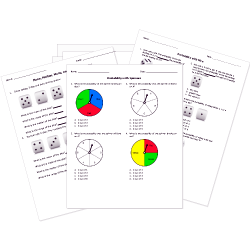Looking for Statistics worksheets?
Check out our pre-made Statistics worksheets!
 Tweet##### Browse Questions

You can create printable tests and worksheets from these Grade 7 Combinations and Permutations questions! Select one or more questions using the checkboxes above each question. Then click the add selected questions to a test button before moving to another page.

Previous Next
The Fundamental Counting Principle
1. uses multiplication to find the number of samples.
2. uses multiplication to find the number of possible outcomes.
3. uses addition to find the number of possible outcomes.
4. uses addition to find the number of samples.
Grade 7 Combinations and Permutations CCSS: 7.SP.C.8, 7.SP.C.8b
How many ways can you arrange the letters in the word TIGER?
1. 5 x 4 x 3 x 2 x 1 = 180
2. 5 x 4 x 3 = 120
3. 5 x 4 x 3 x 2 x 1 = 120
4. 5
Grade 7 Combinations and Permutations CCSS: 7.SP.C.8, 7.SP.C.8b
Previous Next
You need to have at least 5 reputation to vote a question down. Learn How To Earn Badges.